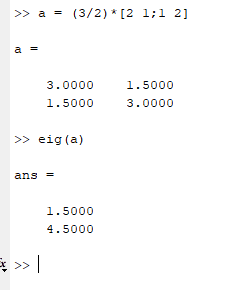# Eigenvalue problem -- Elastic deformation of a membrane

## Homework Statement

An elastic membrane in the x1x2-plane with boundary circle x1^2 + x2^2 = 1 is stretched so that point P(x1,x2) goes over into point Q(y1,y2) such that y = Ax with A = 3/2* [2 1 ; 1 2] find the principal directions and the corresponding factors of extension or contraction of the elastic deformation. Sketch the shape of the deformed membrane.

## Homework Equations

det(A-lambda*I) = 0
(A-lambda*I)x = 0

## The Attempt at a Solution

Using det(A-lambda*I) = 0
I got

lambda = 4.5
lambda = 0

Using (A-lambda*I)x = 0
I got eigenvectors

x2*[1;1]
x2*[-.5;1]

What do I do next?

Last edited by a moderator:

Mark44
Mentor

## Homework Statement

An elastic membrane in the x1x2-plane with boundary circle x1^2 + x2^2 = 1 is stretched so that point P(x1,x2) goes over into point Q(y1,y2) such that y = Ax with A = 3/2* [2 1 ; 1 2] find the principal directions and the corresponding factors of extension or contraction of the elastic deformation. Sketch the shape of the deformed membrane.

## Homework Equations

det(A-lambda*I) = 0
(A-lambda*I)x = 0

## The Attempt at a Solution

Using det(A-lambda*I) = 0
I got

lambda = 4.5
lambda = 0
Check your algebra. Both eigenvalues are incorrect.
shreddinglicks said:
Using (A-lambda*I)x = 0
I got eigenvectors

x2*[1;1]
x2*[-.5;1]
The first eigenvector is correct, although it appears that you got it by accident, not on purpose. The second one isn't correct.
shreddinglicks said:
What do I do next?
After you get the correct eigenvalues and eigenvectors, each eigenvalue will give the amount of stretch or compression in the direction of the associated eigenvector. That should give you some idea of how the circle will be deformed due to the transformation

Yes, it is incorrect. I recalculated.

lambda = 1.5 and 4.5

eigenvectors are:
x2*[-1 1]
x2*[1 1]

I'm confused about the next part. Am I plugging the eigenvalues into x2? Then Am I plugging the vectors into the circle equation x1^2 + x2^2 = 1?

Mark44
Mentor
Yes, it is incorrect. I recalculated.

lambda = 1.5 and 4.5
Those still aren't right. The eigenvalues I get are both integer values. It would be helpful if you showed more of your work, such as the characteristic equation you're working with.

It's a good idea to check your work. If ##\lambda_1## is an eigenvalue with associated eigenvector ##\vec{x_1}##, then ##A\vec{x_1} = \lambda_1\vec{x_1}## should be a true statement. IOW, applying the matrix to an eigenvector should result in a scalar multiple of that eigenvector -- a vector that is longer or shorter or possibly pointing the opposite direction (if the eigenvalue is negative).

I have checked both of my eigenvalues and eigenvectors as described above.
shreddinglicks said:
eigenvectors are:
x2*[-1 1]
x2*[1 1]
These are the eigenvectors I get, but I have no idea how you're getting them, as your eigenvalues are both wrong.
shreddinglicks said:
I'm confused about the next part. Am I plugging the eigenvalues into x2? Then Am I plugging the vectors into the circle equation x1^2 + x2^2 = 1?
The two directions of your eigenvectors define four points on the untransformed circle. Using the correct eigenvalues, the transformed points from the circle will be in the same direction as the eigenvectors, but pushed out or sucked in. The transformation should deform the circle into a rotated ellipse.

I checked my eigenvalues on matlab to be sure.The hand calculation is also attached.

#### Attachments

Yes, multiplied by 3/2.

Mark44
Mentor
OK, my mistake. I wasn't using that factor of 3/2 in my calculations. Since both of your eigenvalues are positive, the points on the circle in the directions of your eigenvectors will be stretched out by factors of 1.5 and 4.5.

I want to understand this. So I have two answers.

One where

x2*[-1 1]' where x2 = 1.5